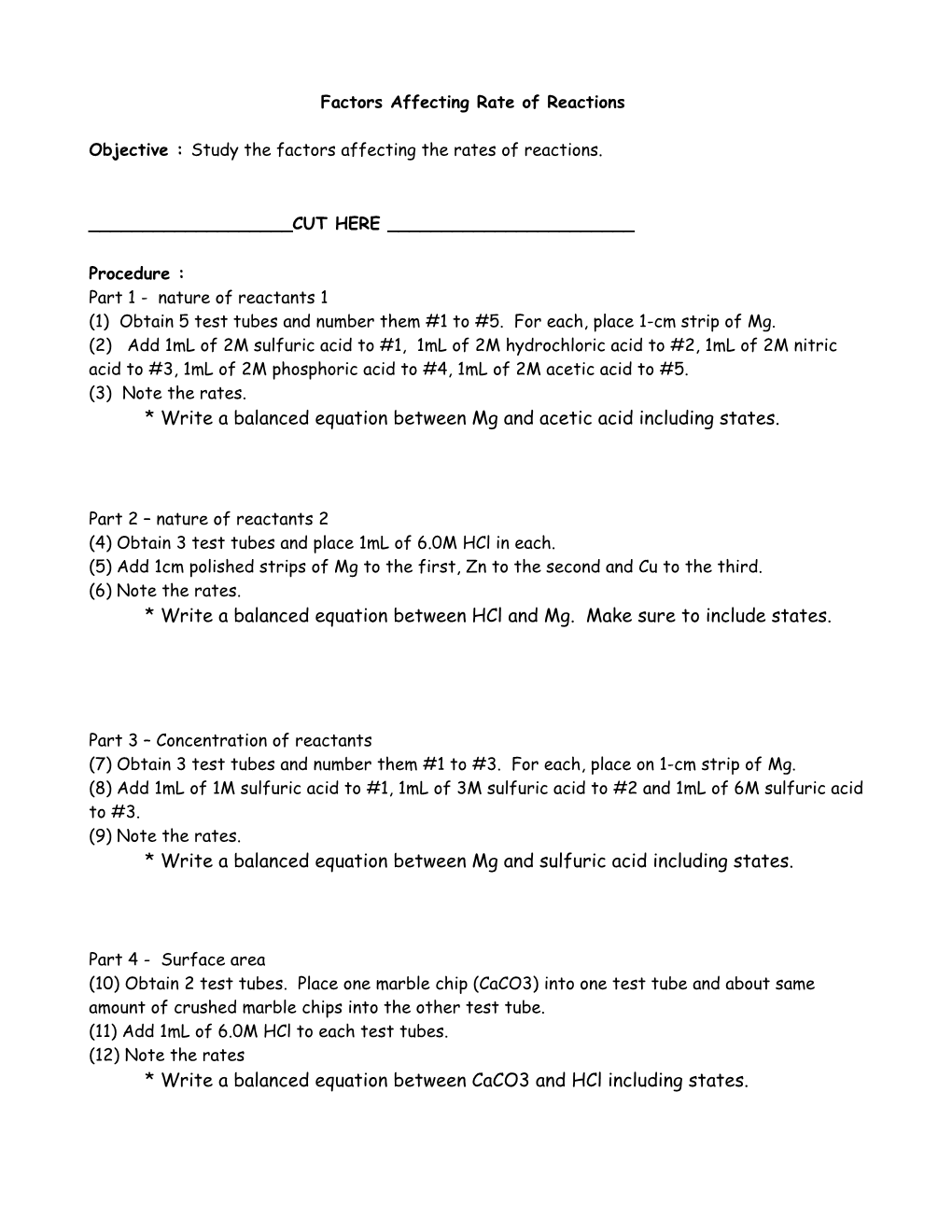# Factors Affecting Rate of ReactionsFactors Affecting Rate of Reactions

Objective :Study the factors affecting the rates of reactions.

______CUT HERE ______

Procedure :

Part 1 - nature of reactants 1

(1) Obtain 5 test tubes and number them #1 to #5. For each, place 1-cm strip of Mg.

(2) Add 1mL of 2M sulfuric acid to #1, 1mL of 2M hydrochloric acid to #2, 1mL of 2M nitric acid to #3, 1mL of 2M phosphoric acid to #4, 1mL of 2M acetic acid to #5.

(3) Note the rates.

* Write a balanced equation between Mg and acetic acid including states.

Part 2 – nature of reactants 2

(4)Obtain 3 test tubes and place 1mL of 6.0M HCl in each.

(5)Add 1cm polished strips of Mg to the first, Zn to the second and Cu to the third.

(6)Note the rates.

* Write a balanced equation between HCl and Mg. Make sure to include states.

Part 3 – Concentration of reactants

(7)Obtain 3 test tubes and number them #1 to #3. For each, place on 1-cm strip of Mg.

(8)Add 1mL of 1M sulfuric acid to #1, 1mL of 3M sulfuric acid to #2 and 1mL of 6M sulfuric acid to #3.

(9)Note the rates.

* Write a balanced equation between Mg and sulfuric acid including states.

Part 4 - Surface area

(10) Obtain 2 test tubes. Place one marble chip (CaCO3) into one test tube and about same amount of crushed marble chips into the other test tube.

(11) Add 1mL of 6.0M HCl to each test tubes.

(12) Note the rates

* Write a balanced equation between CaCO3 and HClincluding states.

Part 5 – Temperature

(13) Obtain 3 test tubes and add 1mL of 6.0M HCl to all three.

(14) Place one test tube in an ice bath, the second in a warm water bath, and the third in a tap water. Wait 5 minutes.

(15) To each test tube, add a piece of Zn.

(16) Note the rates.

* Write a balanced equation between Zn and HClincluding states.

Part 6 – Catalyst

(17) Obtain 2 test tubes and add 1mL of 3% H2O2 to both.

(18) Add few grains of MnO2 to one of the test tubes.

(19) Note the rates.

* Write a balanced equation for decomposition of H2O2including states.

______CUT HERE ______

Prelab Questions :

(PL1) List some factors that affect the rate of reactions.

(PL2) Rate is quantitative with unit of M/sec. We are not measuring anything quantitative for this lab. How would you study the rate for this lab?

Postlab Questions:

(Q1) How did you note the rate for each part? i.e. Color changing to red? Test tube getting hot?

(Q2) What would happen to the rate in part 1, if you use cubic chunks of Mg instead of Mg ribbon of same amount? Why?

Summary :

For each part, list the reactions in increasing rate.

For example,

for part 2, Mg + HCl < Cu + HCl < Zn + HCl (Zn being the fastest reaction)

“Mg < Cu < Zn” is not complete!